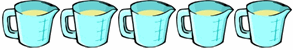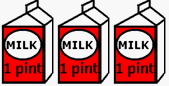Skill 27A Measurement: Cups, Pints, Quarts Volume measurement of liquids is perhaps the most difficult measurement concept for students to master.  To answer the comparison questions here, your student will need to know how many cups are in a pint and how many pints are in a quart (by the way, I'm sure all of you know the answer, but there are 2 cups in a pint and 2 pints in a quart). Although this skill is simple enough that it should be able to be answered without separate calculations on paper (looking at the screen should be adequate), some students may need the help of paper and pencil.  If they do, it shouldn't take longer than a minute to find the answer, otherwise the skill should be practiced. Which group of containers holds more? Is it the two pints of milk or the 5 blue containers that hold one cup each? You can use a paper and pencil to help find the answer if you would like.each container holds 1 pint each container holds 1 cup In this next problem we have one quart of milk on the left and 3 single pints of milk on the right. Which of these hold more?  Again, you can use a paper and pencil to help find the answer if you would like.1 quart each container holds 1 pint